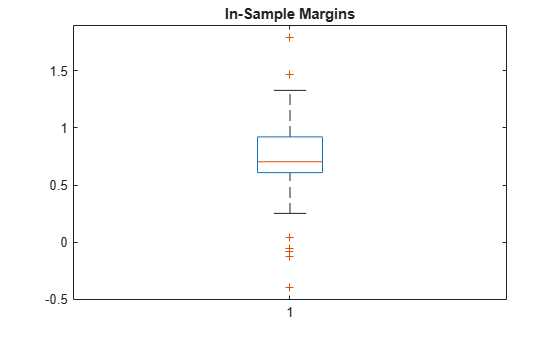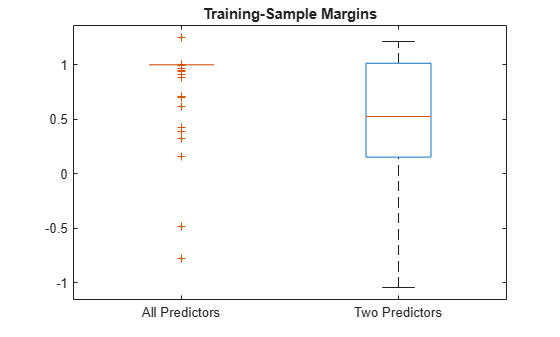Documentation

# resubMargin

Resubstitution classification margins for multiclass error-correcting output codes (ECOC) model

## Syntax

``m = resubMargin(Mdl)``
``m = resubMargin(Mdl,Name,Value)``

## Description

example

````m = resubMargin(Mdl)` returns the resubstitution classification margins (`m`) for the multiclass error-correcting output codes (ECOC) model `Mdl` using the training data stored in `Mdl.X` and the corresponding class labels stored in `Mdl.Y`.`m` is returned as a numeric column vector with the same length as `Mdl.Y`. The software estimates each entry of `m` using the trained ECOC model `Mdl`, the corresponding row of `Mdl.X`, and the true class label `Mdl.Y`.```
````m = resubMargin(Mdl,Name,Value)` returns the classification margins with additional options specified by one or more name-value pair arguments. For example, you can specify a decoding scheme, binary learner loss function, and verbosity level.```

## Examples

collapse all

Calculate the resubstitution classification margins for an ECOC model with SVM binary learners.

Load Fisher's iris data set. Specify the predictor data `X` and the response data `Y`.

```load fisheriris X = meas; Y = species;```

Train an ECOC model using SVM binary classifiers. Standardize the predictors using an SVM template, and specify the class order.

```t = templateSVM('Standardize',true); classOrder = unique(Y)```
```classOrder = 3x1 cell array {'setosa' } {'versicolor'} {'virginica' } ```
`Mdl = fitcecoc(X,Y,'Learners',t,'ClassNames',classOrder);`

`t` is an SVM template object. During training, the software uses default values for empty properties in `t`. `Mdl` is a `ClassificationECOC` model.

Calculate the classification margins for the observations used to train `Mdl`. Display the distribution of the margins using a boxplot.

```m = resubMargin(Mdl); boxplot(m) title('In-Sample Margins')```The classification margin of an observation is the positive-class negated loss minus the maximum negative-class negated loss. Choose classifiers that yield relatively large margins.

Perform feature selection by comparing training-sample margins from multiple models. Based solely on this comparison, the model with the greatest margins is the best model.

Load Fisher's iris data set. Define two data sets:

• `fullX` contains all four predictors.

• `partX` contains the sepal measurements only.

```load fisheriris X = meas; fullX = X; partX = X(:,1:2); Y = species;```

Train an ECOC model using SVM binary learners for each predictor set. Standardize the predictors using an SVM template, specify the class order, and compute posterior probabilities.

```t = templateSVM('Standardize',true); classOrder = unique(Y)```
```classOrder = 3x1 cell array {'setosa' } {'versicolor'} {'virginica' } ```
```FullMdl = fitcecoc(fullX,Y,'Learners',t,'ClassNames',classOrder,... 'FitPosterior',true); PartMdl = fitcecoc(partX,Y,'Learners',t,'ClassNames',classOrder,... 'FitPosterior',true);```

Compute the resubstitution margins for each classifier. For each model, display the distribution of the margins using a boxplot.

```fullMargins = resubMargin(FullMdl); partMargins = resubMargin(PartMdl); boxplot([fullMargins partMargins],'Labels',{'All Predictors','Two Predictors'}) title('Training-Sample Margins')```The margin distribution of `FullMdl` is situated higher and has less variability than the margin distribution of `PartMdl`. This result suggests that the model trained with all the predictors fits the training data better.

## Input Arguments

collapse all

Full, trained multiclass ECOC model, specified as a `ClassificationECOC` model trained with `fitcecoc`.

### Name-Value Pair Arguments

Specify optional comma-separated pairs of `Name,Value` arguments. `Name` is the argument name and `Value` is the corresponding value. `Name` must appear inside quotes. You can specify several name and value pair arguments in any order as `Name1,Value1,...,NameN,ValueN`.

Example: `resubMargin(Mdl,'Verbose',1)` specifies to display diagnostic messages in the Command Window.

Binary learner loss function, specified as the comma-separated pair consisting of `'BinaryLoss'` and a built-in loss function name or function handle.

• This table describes the built-in functions, where yj is a class label for a particular binary learner (in the set {–1,1,0}), sj is the score for observation j, and g(yj,sj) is the binary loss formula.

ValueDescriptionScore Domaing(yj,sj)
`'binodeviance'`Binomial deviance(–∞,∞)log[1 + exp(–2yjsj)]/[2log(2)]
`'exponential'`Exponential(–∞,∞)exp(–yjsj)/2
`'hamming'`Hamming[0,1] or (–∞,∞)[1 – sign(yjsj)]/2
`'hinge'`Hinge(–∞,∞)max(0,1 – yjsj)/2
`'linear'`Linear(–∞,∞)(1 – yjsj)/2
`'logit'`Logistic(–∞,∞)log[1 + exp(–yjsj)]/[2log(2)]
`'quadratic'`Quadratic[0,1][1 – yj(2sj – 1)]2/2

The software normalizes binary losses so that the loss is 0.5 when yj = 0. Also, the software calculates the mean binary loss for each class.

• For a custom binary loss function, for example `customFunction`, specify its function handle `'BinaryLoss',@customFunction`.

`customFunction` has this form:

`bLoss = customFunction(M,s)`
where:

• `M` is the K-by-L coding matrix stored in `Mdl.CodingMatrix`.

• `s` is the 1-by-L row vector of classification scores.

• `bLoss` is the classification loss. This scalar aggregates the binary losses for every learner in a particular class. For example, you can use the mean binary loss to aggregate the loss over the learners for each class.

• K is the number of classes.

• L is the number of binary learners.

For an example of passing a custom binary loss function, see Predict Test-Sample Labels of ECOC Model Using Custom Binary Loss Function.

The default `BinaryLoss` value depends on the score ranges returned by the binary learners. This table describes some default `BinaryLoss` values based on the given assumptions.

AssumptionDefault Value
All binary learners are SVMs or either linear or kernel classification models of SVM learners.`'hinge'`
All binary learners are ensembles trained by `AdaboostM1` or `GentleBoost`.`'exponential'`
All binary learners are ensembles trained by `LogitBoost`.`'binodeviance'`
All binary learners are linear or kernel classification models of logistic regression learners. Or, you specify to predict class posterior probabilities by setting `'FitPosterior',true` in `fitcecoc`.`'quadratic'`

To check the default value, use dot notation to display the `BinaryLoss` property of the trained model at the command line.

Example: `'BinaryLoss','binodeviance'`

Data Types: `char` | `string` | `function_handle`

Decoding scheme that aggregates the binary losses, specified as the comma-separated pair consisting of `'Decoding'` and `'lossweighted'` or `'lossbased'`. For more information, see Binary Loss.

Example: `'Decoding','lossbased'`

Estimation options, specified as the comma-separated pair consisting of `'Options'` and a structure array returned by `statset`.

To invoke parallel computing:

• You need a Parallel Computing Toolbox™ license.

• Specify `'Options',statset('UseParallel',true)`.

Verbosity level, specified as the comma-separated pair consisting of `'Verbose'` and `0` or `1`. `Verbose` controls the number of diagnostic messages that the software displays in the Command Window.

If `Verbose` is `0`, then the software does not display diagnostic messages. Otherwise, the software displays diagnostic messages.

Example: `'Verbose',1`

Data Types: `single` | `double`

collapse all

### Classification Margin

The classification margin is, for each observation, the difference between the negative loss for the true class and the maximal negative loss among the false classes. If the margins are on the same scale, then they serve as a classification confidence measure. Among multiple classifiers, those that yield greater margins are better.

### Binary Loss

A binary loss is a function of the class and classification score that determines how well a binary learner classifies an observation into the class.

Suppose the following:

• mkj is element (k,j) of the coding design matrix M (that is, the code corresponding to class k of binary learner j).

• sj is the score of binary learner j for an observation.

• g is the binary loss function.

• $\stackrel{^}{k}$ is the predicted class for the observation.

In loss-based decoding [Escalera et al.], the class producing the minimum sum of the binary losses over binary learners determines the predicted class of an observation, that is,

`$\stackrel{^}{k}=\underset{k}{\text{argmin}}\sum _{j=1}^{L}|{m}_{kj}|g\left({m}_{kj},{s}_{j}\right).$`

In loss-weighted decoding [Escalera et al.], the class producing the minimum average of the binary losses over binary learners determines the predicted class of an observation, that is,

`$\stackrel{^}{k}=\underset{k}{\text{argmin}}\frac{\sum _{j=1}^{L}|{m}_{kj}|g\left({m}_{kj},{s}_{j}\right)}{\sum _{j=1}^{L}|{m}_{kj}|}.$`

Allwein et al. suggest that loss-weighted decoding improves classification accuracy by keeping loss values for all classes in the same dynamic range.

This table summarizes the supported loss functions, where yj is a class label for a particular binary learner (in the set {–1,1,0}), sj is the score for observation j, and g(yj,sj).

ValueDescriptionScore Domaing(yj,sj)
`'binodeviance'`Binomial deviance(–∞,∞)log[1 + exp(–2yjsj)]/[2log(2)]
`'exponential'`Exponential(–∞,∞)exp(–yjsj)/2
`'hamming'`Hamming[0,1] or (–∞,∞)[1 – sign(yjsj)]/2
`'hinge'`Hinge(–∞,∞)max(0,1 – yjsj)/2
`'linear'`Linear(–∞,∞)(1 – yjsj)/2
`'logit'`Logistic(–∞,∞)log[1 + exp(–yjsj)]/[2log(2)]
`'quadratic'`Quadratic[0,1][1 – yj(2sj – 1)]2/2

The software normalizes binary losses such that the loss is 0.5 when yj = 0, and aggregates using the average of the binary learners [Allwein et al.].

Do not confuse the binary loss with the overall classification loss (specified by the `'LossFun'` name-value pair argument of the `loss` and `predict` object functions), which measures how well an ECOC classifier performs as a whole.

## Tips

• To compare the margins or edges of several ECOC classifiers, use template objects to specify a common score transform function among the classifiers during training.

 Allwein, E., R. Schapire, and Y. Singer. “Reducing multiclass to binary: A unifying approach for margin classiﬁers.” Journal of Machine Learning Research. Vol. 1, 2000, pp. 113–141.

 Escalera, S., O. Pujol, and P. Radeva. “On the decoding process in ternary error-correcting output codes.” IEEE Transactions on Pattern Analysis and Machine Intelligence. Vol. 32, Issue 7, 2010, pp. 120–134.

 Escalera, S., O. Pujol, and P. Radeva. “Separability of ternary codes for sparse designs of error-correcting output codes.” Pattern Recogn. Vol. 30, Issue 3, 2009, pp. 285–297.